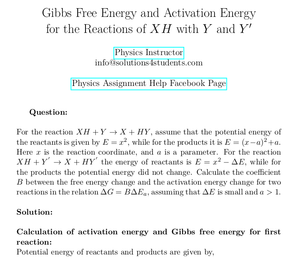Solved Problems in Physics, Physical Chemistry, and Computer Simulation Projects for College and Graduate Students.# Gibbs Free Energy and Activation Energy for the Reactions of XH with Y and Y'

Regular price
\$16.50
Sale price
\$16.50
Tax included.

Question:
For the reaction XH + Y → X + HY , assume that the potential energy of the reactants is given by E = x2 , while for the products it is E = (x−a)2 +a. Here x is the reaction coordinate, and a is a parameter. For the reaction XH + Y' → X + HY' the energy of reactants is E = x2 − ∆E, while for the products the potential energy did not change. Calculate the coefficient B between the free energy change and the activation energy change for two reactions in the relation ∆G = B∆Ea , assuming that ∆E is small and a > 1.

By purchasing this product, you will get the step by step solution of the above problem in pdf format and the corresponding latex file where you can edit the solution. I am also available to help  you with any possible question you may have.